# Relativistic Energy

The famous Einstein relationship for energyincludes both the kinetic energy and rest mass energy for a particle. The kinetic energy of a high speed particle can be calculated fromThe relativistic energy of a particle can also be expressed in terms of its momentum in the expression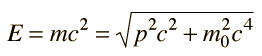Show

The relativistic energy expression is the tool used to calculate binding energies of nuclei and the energy yields of nuclear fission and fusion.

Index

 HyperPhysics***** Relativity R Nave
Go Back

# Rest Mass Energy

The Einstein equation includes both the kinetic energy of a particle and the energy it has as a result of its mass. If the particle is at rest, then the energy is expressed as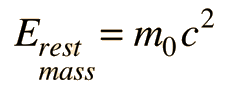which is sometimes called its rest mass energy.

 For rest mass m0 = me =mp =x10^kg

 where me= electron rest mass and mp= proton rest mass

then the rest mass energy is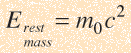= eV =MeV = GeV = x10^joules

 Energy application Weapon application
Index

 HyperPhysics***** Relativity R Nave
Go Back

# Conservation of Energy

The relativistic energy expression E = mc2 is a statement about the energy an object contains as a result of its mass and is not to be construed as an exception to the principle of conservation of energy. Energy can exist in many forms, and mass energy can be considered to be one of those forms.

"Energy is the ultimate convertable currency."
(Brian Greene in The Elegant Universe)

Index

 HyperPhysics***** Relativity R Nave
Go Back

# Pair Production

Every known particle has an antiparticle; if they encounter one another, they will annihilate with the production of two gamma-rays. The quantum energies of the gamma rays is equal to the sum of the mass energies of the two particles (including their kinetic energies). It is also possible for a photon to give up its quantum energy to the formation of a particle-antiparticle pair in its interaction with matter.

The rest mass energy of an electron is 0.511 MeV, so the threshold for electron-positron pair production is 1.02 MeV. For x-ray and gamma-ray energies well above 1 MeV, this pair production becomes one of the most important kinds of interactions with matter. At even higher energies, many types of particle-antiparticle pairs are produced.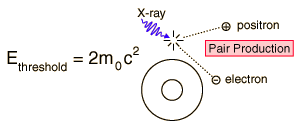Index

 HyperPhysics***** Relativity R Nave
Go Back

# Relativistic Kinetic Energy

The relativistic energy expression includes both rest mass energy and the kinetic energy of motion. The kinetic energy is then given byThis is essentially defining the kinetic energy of a particle as the excess of the particle energy over its rest mass energy. For low velocities this expression approaches the non-relativistic kinetic energy expression.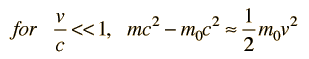Show
 For a mass of m =x10^kg = me = mp

 with mass energy m0c2 = x10^eV/c2 =MeV/c2 = GeV/c2

 traveling at velocity v = x10^ m/s = c, gamma = γ =

the kinetic energy is

K.E.(relativistic) =x10^ joules = x10^ eV.
This compares to a non-relativistic result of x10^ joules ,
which would be in error by %.

 Relativistic Mechanical Quantities
Index

 HyperPhysics***** Relativity R Nave
Go Back

# Kinetic Energy for v/c<<1

The relativistic kinetic energy expression can be written as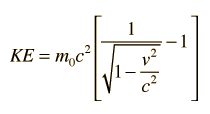and the square root expression then expanded by use of the binomial theorem :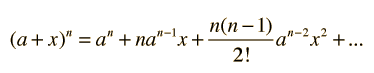giving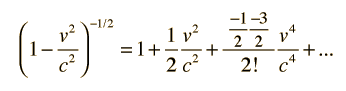Substituting gives: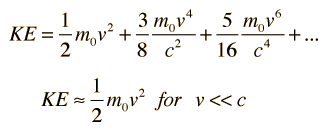Index

 HyperPhysics***** Relativity R Nave
Go Back